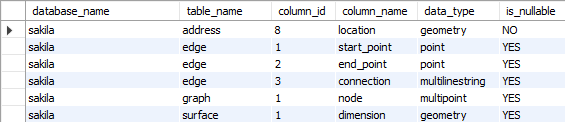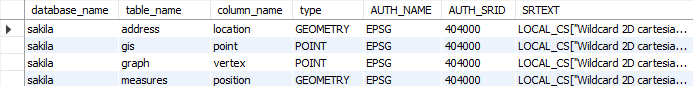## Find all spatial data columns in MariaDB database

Article for:

Spatial in MariaDB are columns with the following data types: geometry, point, linestring, polygon, multipoint, multilinestring, multipolygon, geometrycollection

## Note

If you are using MariaDB 10.1.2 or newer, you can use query obtaining spatial references system: MariaDB 10.1.2+ Query

## Universal

The query below lists all columns with spatial data types.

### Query

``````select col.table_schema as database_name,
col.table_name,
col.ordinal_position as column_id,
col.column_name,
col.data_type,
col.is_nullable
from information_schema.columns col
join information_schema.tables tab
on col.table_schema = tab.table_schema
and col.table_name = tab.table_name
and table_type = 'BASE TABLE'
where col.data_type in ('geometry', 'point', 'linestring', 'polygon',
'multipoint', 'multilinestring', 'multipolygon',
'geometrycollection')
and col.table_schema not in ('information_schema', 'sys',
'performance_schema', 'mysql')
-- and table_schema = 'database_name' -- put your database name here
order by col.table_schema,
col.table_name;
``````

### Columns

• database_name - name of the database (schema)
• table_name - name of the table
• column_id - column position in table
• column_name - name of the column
• data_type - type of data
• GEOMETRY
• POINT
• LINESTRING
• POLYGON
• MULTIPOINT
• MULTILINESTRING
• MULTIPOLYGON
• GEOMETRYCOLLECTION
• is_nullable - indicate whether column can contains null values

### Rows

• One row represents one column with a spatial data type
• Scope of rows: all columns containing spatial data types in the database (schema)
• Ordered by database name and table name

### Sample resultsIn this version of MariaDB introduced GEOMETRY_COLUMN and SPATIAL_REF_SYS tables.

### Query

``````select g_table_schema as database_name,
g_table_name as table_name,
g_geometry_column as column_name,
case geometry_type
when 0 then 'GEOMETRY'
when 1 then 'POINT'
when 2 then 'LINESTRING'
when 3 then 'POLYGON'
when 4 then 'MULTIPOINT'
when 5 then 'MULTILINESTRING'
when 6 then 'MULTIPOLYGON'
when 7 then 'GEOMETRYCOLLECTION'
end as type,
auth_name,
auth_srid,
srtext
from information_schema.geometry_columns col
join information_schema.spatial_ref_sys ref
on col.srid = ref.srid
order by g_table_schema,
g_table_name;
``````

### Columns

• database_name - name of the database (schema)
• table_name - name of the table
• column_name - name of the column
• type - geometry type of data
• GEOMETRY
• POINT
• LINESTRING
• POLYGON
• MULTIPOINT
• MULTILINESTRING
• MULTIPOLYGON
• GEOMETRYCOLLECTION
• auth_name - name of the standard or standards body that is being cited for reference system
• auth_srid - ID of the coordinate system in the above authority's catalog.
• srtext - Well-known Text Representation of the Spatial Reference System

### Rows

• One row represents one column with a spatial data type
• Scope of rows: all columns containing spatial data types in the database (schema)
• Ordered by database name and table name

### Sample results